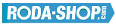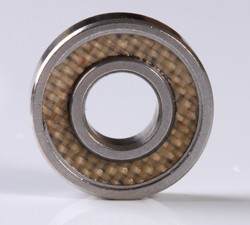# Remote ControlManufacturer Product Image Item Name Price+### 30/5-2Z

Miniature Metric Series Chrome Steel Metallic Shields on both sides Dimension d=5.00 Dimension D=14.00 Dimension B=7.00 Dinamic Load Rating=2020 Static Load Rating=930 Weight=0.008gr. Speed limit Grease=30.0 Speed limit Oil=38.0
inside diameter = 5.00
outside diameter = 14.00
width = 7.00### 30/7-2RS

Miniature Metric Series Chrome Steel Rubber Seals on Both sides Dimension d=7.00 Dimension D=19.00 Dimension B=10.00 Dinamic Load Rating=3650 Static Load Rating=2000 Weight=0.012gr. Speed limit Grease=28.0 Speed limit Oil=30.0
inside diameter = 7.00
outside diameter = 19.00
width = 10.00### 30/7-2Z

Miniature Metric Series Chrome Steel Metallic Shields on both sides Dimension d=7.00 Dimension D=19.00 Dimension B=10.00 Dinamic Load Rating=3650 Static Load Rating=2000 Weight=0.012gr. Speed limit Grease=28.0 Speed limit Oil=30.0
inside diameter = 7.00
outside diameter = 19.00
width = 10.00### 30/8-2Z

Miniature Metric Series Chrome Steel Metallic Shields on both sides Dimension d=8.00 Dimension D=22.00 Dimension B=11.00 Dinamic Load Rating=5070 Static Load Rating=2580 Weight=0.02gr. Speed limit Grease=30.0 Speed limit Oil=36.0
inside diameter = 8.00
outside diameter = 22.00
width = 11.00### 62800

Thin Section Bearings Chrome Steel Open(No Shields) Dimension d=10.00 Dimension D=19.00 Dimension B=6.00 Dinamic Load Rating=0 Static Load Rating=0 Weight=0.007gr. Speed limit Grease=0.0 Speed limit Oil=-
inside diameter = 10.00
outside diameter = 19.00
width = 6.00### 62800-2Z

Thin Section Bearings Chrome Steel Metallic Shields on both sides Dimension d=10.00 Dimension D=19.00 Dimension B=6.00 Dinamic Load Rating=0 Static Load Rating=0 Weight=0.007gr. Speed limit Grease=0.0 Speed limit Oil=-
inside diameter = 10.00
outside diameter = 19.00
width = 6.00### 63801-2Z

Thin Section Bearings Chrome Steel Metallic Shields on both sides Dimension d=12.00 Dimension D=21.00 Dimension B=7.00 Dinamic Load Rating=1915 Static Load Rating=1041 Weight=0.01gr. Speed limit Grease=33.0 Speed limit Oil=39.0
inside diameter = 12.00
outside diameter = 21.00
width = 7.00### 63802-2Z

Thin Section Bearings Chrome Steel Metallic Shields on both sides Dimension d=15.00 Dimension D=24.00 Dimension B=7.00 Dinamic Load Rating=2073 Static Load Rating=1253 Weight=0.011gr. Speed limit Grease=28.0 Speed limit Oil=33.0
inside diameter = 15.00
outside diameter = 24.00
width = 7.00### 683-2TS

Miniature Metric Series Chrome Steel PTFE Seales on both sides Dimension d=3.00 Dimension D=7.00 Dimension B=3.00 Dinamic Load Rating=311 Static Load Rating=112 Weight=0.001gr. Speed limit Grease=63.0 Speed limit Oil=75.0
inside diameter = 3.00
outside diameter = 7.00
width = 3.00### 686-2TS4.5

Miniature Metric Series Chrome Steel PTFE Seales on both sides Dimension d=6.00 Dimension D=13.00 Dimension B=4.50 Dinamic Load Rating=1082 Static Load Rating=442 Weight=0.004gr. Speed limit Grease=40.0 Speed limit Oil=50.0
inside diameter = 6.00
outside diameter = 13.00
width = 4.50